##Involutive homographies on conics

In InvolutiveHomography.html we saw that such a map H on the points of a conic (c) defines a particular point F (Fregier point) which is the pol of the corresponding homography axis. We noticed further that H extends to a harmonic perspectivity H0 which is a global projectivity. Here we mention two particular families of conics whose members remain invariant under H0, hence introduce corresponding involutive homographies on these.
 The first (I) is the family generated by the conic (c) and its Fregier point (A).
 The second (II) is the family generated by the conic (c) and its homography axis.
In both cases all conics of the family have A and the axis as pol/polar. This shows that two homographies on two conics can give the same extension to a global projectivity on the whole projective plane.
A natural question that may arise is the one of determination of all conics (c) having a given point A and a given line (e) as Fregier point/homography axis respectively. Equivalently all conics invariant under the harmonic perspectivity H0. The previous remarks give a partial answer:
 Bundle (II) is determined by A and the two points B, C on the axis. Taking B and C arbitrary on this axis we can define the corresponding bitangent bundle of conics, whose members pass all through B, C and have there tangents the lines BA, CA correspondingly (see BitangentConics.html ).
 Each member of a bundle of type (II) together with point (A) generate a family of conics of type (I) also invariant by the harmonic perspectivity defined by A and the original axis. Further (I) does not depend on the particula member of the bundle (II) used for its definition (all members of the same bundle defining the same bundle of type (I)).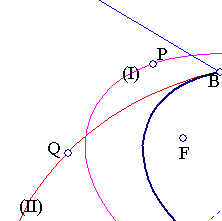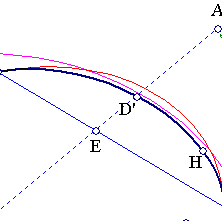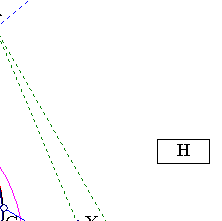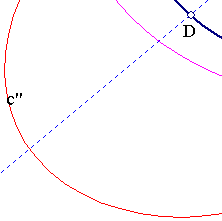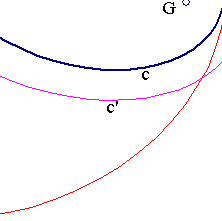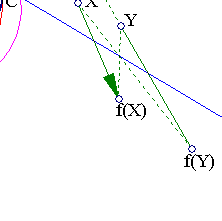There is also a circle bundle which has many members invariant under the aforementioned perspectivity. This is the circle bundle having one limit point A and radical axis the parallel to (e) at half distance from it. The circles of this bundle containing point A remain also invariant under H0. These circles though are contained (each in a different) in the bundles of type (I). So they represent no real addition to  and . I have not yet a full proof that these are the only solutions, but it seems very likely it is so. For this it would suffice to show that all conics invariant under H0 and not intersecting line (e) belong to some bundle of type (I).